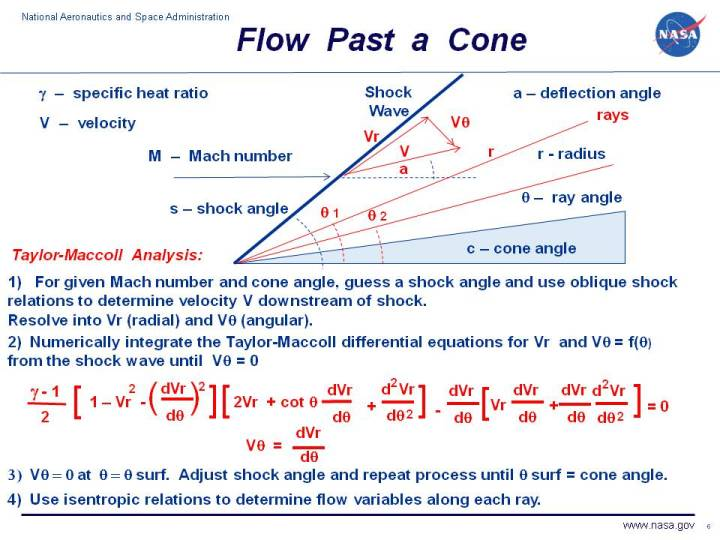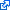+ Text Only Site
+ Non-Flash Version
+ Contact GlennAs an object moves through a gas, the gas molecules are deflected around the object. If the speed of the object is much less than the speed of sound of the gas, the density of the gas remains constant and the flow of gas can be described by conserving momentum, and energy. As the speed of the object approaches the speed of sound, we must consider compressibility effects on the gas. The density of the gas varies locally as the gas is compressed by the object. For compressible flows with little or small flow turning, the flow process is reversible and the entropy is constant. The change in flow properties are then given by the isentropic relations (isentropic means "constant entropy"). But when an object moves faster than the speed of sound, and there is an abrupt decrease in the flow area, shock waves are generated in the flow. Shock waves are very small regions in the gas where the gas properties change by a large amount. Across a shock wave, the static pressure, temperature, and gas density increases almost instantaneously. The changes in the flow properties are irreversible and the entropy of the entire system increases. Because a shock wave does no work, and there is no heat addition, the total enthalpy and the total temperature are constant. But because the flow is non-isentropic, the total pressure downstream of the shock is always less than the total pressure upstream of the shock. There is a loss of total pressure associated with a shock wave. On this web page, we show a method for determining the supersonic flow past a cone. The method was first developed by G.I. Taylor and J.W. Maccoll in 1933. The derivation of the differential equation shown on the slide is fairly complicated. The method assumes that the supersonic flow along a cone is simplified because of symmetry considerations. We define a polar coordinate system through the point of the cone, with r being the radial coordinate along rays that meet at the point, theta being the angle that the ray makes with a reference line through the axis of the cone, and phi being the angle measured around the axis. All changes with phi are equal to zero because the flow is axisymmetric. d (variable) / d phi = 0 where d represents a partial derivitive. On the figure we show a two dimensional cut at any representative value of phi. The analysis can be further simplified by assuming that the flow variables do not change with r; flow values are constant along a ray and therefore only vary with theta d (variable) / d r = 0 By taking the conservation of mass. momentum, and energy equations, written in polar coordinates, and applying the simplifications given above, Taylor and Maccoll were able to derive the differential equations given on the slide which express the change in flow variables with theta from behind the oblique shock to the surface of the cone. [(gamma -1) /2][1 - Vr^2 - (d Vr / d theta)^2][2Vr + cot(theta)d Vr / d theta + d^2 Vr / d theta^2] - d Vr / d theta * [Vr * d Vr / d theta + d Vr / d theta * d^2 Vr / d theta^2] = 0 Vtheta = d Vr / d theta where gamma is the ratio of specific heats, Vr is the radial component of the velocity, and Vtheta is the angular velocity component perpendicular to the rays. d Vr / d theta is an ordinary derivitive, since the radial velocity varies only with theta. There is no known closed solution to this ordinary differential equation, so the solution must be obtained by numerical analysis. The Taylor-Maccoll analysis proceeds as follows. With a known free stream mach number M and known cone angle c, assume a value for the obique shock angle s. The oblique shock relations provides the values of change in flow variables across the shock, and the deflection a of the flow through the shock. Determine the velocity components Vr and Vtheta immediately downstream of the shock. The differential equation can be evaluated by selecting some incremental change in theta and integrating using a fourth order Runge-Kutta scheme from the shock until the value of Vtheta is equal to zero. Vtheta = 0 is the normal velocity condition at the surface of the cone. Knowing the value of theta = theta surface for which Vtheta = 0 and the initial guess of the shock angle s, gives a solution of the Taylor-Maccoll differential equation. A cone of angle theta surface generates a shock of angle s. In general, theta surface will not be equal to the desired cone angle c. Modify the assumed shock angle and repeat the solution of the differential equation to obtain a new pair of shock angle s and theta surface. Repeat this procedure until theta surface = cone angle c. Then the resulting shock angle s is the desired result for cone angle c. In determining the shock angle, the variation of flow velocity and Mach number from the shock to the cone surface is also determined. The isentropic flow relations can then be used to determine the value of the flow variables from the shock to the cone surface. Here's a Java program that performs the Taylor-Maccoll analysis. You can use this simulator to study the flow past a cone. Due to IT security concerns, many users are currently experiencing problems running NASA Glenn educational applets. The applets are slowly being updated, but it is a lengthy process. If you are familiar with Java Runtime Environments (JRE), you may want to try downloading the applet and running it on an Integrated Development Environment (IDE) such as Netbeans or Eclipse. The following are tutorials for running Java applets on either IDE: NetbeansEclipseThis page shows an interactive Java applet for supersonic flow past a cone. The applet shows the shock wave generated by the cone and the value of the flow variables downstream of the shock. Input to the program can be made using the sliders, or input boxes at the upper right. To change the value of an input variable, simply move the slider. Or click on the input box, select and replace the old value, and hit Enter to send the new value to the program. Output from the program is displayed in output boxes at the lower right. The flow variables are constant along rays from the leading edge of the cone. To display the rays. use the drop menu at the lower part of the output panel next to the word "Ray Plot". To select a given ray and display the value of the flow variables along the ray, use the drop menu at the upper part of the output panel next to the word "Ray". Variables are presented as ratios to free stream values. The graphic at the left shows the cone (in red) and the shock wave generated by the cone as a line. The line is colored blue for an oblique shock and magenta when the shock is a normal shock. The black lines show the streamlines of the flow past the cone. Notice that downstream (to the right) of the shock wave, the lines are curved as the velocity components vary along the rays. Downstream, the streamlines are closer together than upstream which indicates an increase in the density of the flow. There is more complete shock simulation program that is also avaliable at this web site. It solves for both flow past a wedge and flow past a cone. If you are an experienced user of the simulator, you can use a sleek version of the program which loads faster on your computer and does not include the user's manual. The ShockModeler program models the intersection and reflection of multiple oblique shock waves generated by multiple wedges. You can also download your own copy of the program to run off-line by clicking on this button: Activities: Guided ToursCompressible Aerodynamics:Shock Waves and Expansions:Single Shock Wave Simulator:Multiple Shock Wave Simulator:Sources of Drag:Inlet:Navigation ..Beginner's Guide Home Page+ Inspector General Hotline + Equal Employment Opportunity Data Posted Pursuant to the No Fear Act + Budgets, Strategic Plans and Accountability Reports + Freedom of Information Act + The President's Management Agenda + NASA Privacy Statement, Disclaimer, and Accessibility CertificationEditor: Nancy Hall NASA Official: Nancy Hall Last Updated: May 13 2021 + Contact Glenn